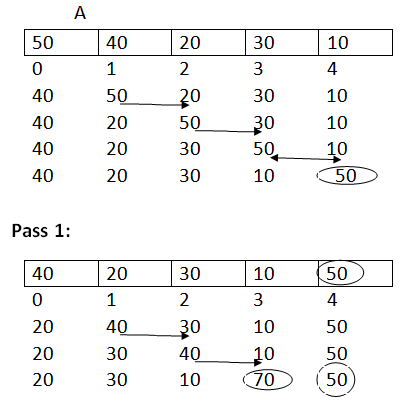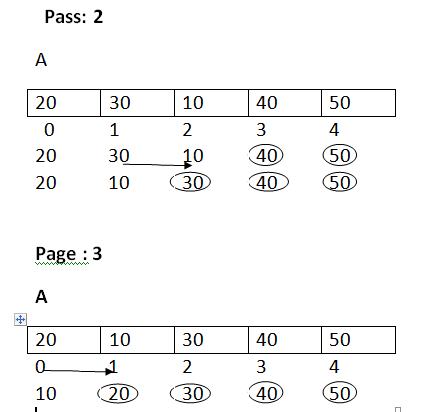# Bubble Sort

Blog Author

Tekslate

Published Date

21st September, 2018

Ratings

Views

125

Bubble Sort The bubble sort is the oldest and simplest sort in use unfortunately, it is also the slowest.

1. compare two numbers at a time, starting with the first two numbers
2. If the first number, is larger than second number, than exchange the two numbers,
3. Go right one number and compare that number to the number that follows it. The two form a new pair.
4. Continue this process until no exchange has been made in an entire pass through the list.

Ex : Pass: 0### Implementation of Bubble sort

# include<stdio.h> void bubble sort(int A,int n); void main() { int A,n,i; printf(“Enter n value \n”); scanf(“%d”, &n); printf(“Enter the elements:\n”); for(i=0;i<n; i++) { scanf(“%d”,&A[i]); } bubble sort[A,n]; printf(“The following are the elements in sorting orfer:\n”); for(i=0;i<n;i++) { printf(“%5d”,a[i]); } } void bubble sort(int A,int n) { int pass, j,temp; for(pass=0;pass<n-1;pass++) { for(j=0;j<n-pass-1;j++) { if(a[j]>a[j+1]) { temp=A[j]; A[j]=A[j+1]; A[j+1]=temp; } } } } Enter n value: 10 Enter the elements : 5 4 1 6 7 9 2 3 8     The following are the elements in the sorting order : 1       2       3       4       5       6       7       8       9       10

## Time complexity:

Traditionally, the time for a sorting algorithm is measured in terms of the number of comparisons specially, There are n-1 comparisons during the first pass, which phases the largest element in the last position, these are n-2 comparisons in second step, which places the second largest element in the next-to-last position, and so on. Thus f(n)=(n-1) + (n-2) +(n-3) + -------------- +2+1 =n(n-1)/2=n2/2-n/2=0(n2)